# Completely-integrable differential equation

Jump to: navigation, search

An equation of the form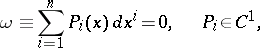(*)

for which an-dimensional integral manifold passes through each point of a certain domain in the space. A necessary and sufficient condition for complete integrability of the differential equation (*) is the Frobenius condition, whereis the symbol of the exterior product . If, this condition has the form: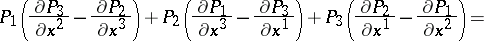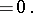Instead of equation (*) the following system of equations is sometimes considered :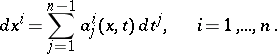In this case the conditions of complete integrability assume the form: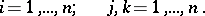The family of integral manifolds of a completely-integrable differential equation is a foliation .